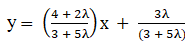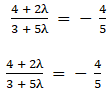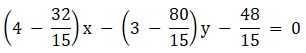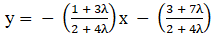• +91 9971497814
• info@interviewmaterial.com

# RD Chapter 23- The Straight Lines Ex-23.19 Interview Questions Answers

### Related Subjects

Question 1 : Find the equation of a straight line through the point of intersection of the lines 4x – 3y = 0 and 2x – 5y + 3 = 0 and parallel to 4x + 5 y + 6 = 0.

Given:

Lines 4x – 3y = 0 and2x – 5y + 3 = 0 and parallel to 4x + 5 y + 6 = 0

The equation of thestraight line passing through the points of intersection of 4x − 3y =0 and 2x − 5y + 3 = 0 is given below:

4x − 3y+ λ (2x − 5y + 3) = 0

(4 + 2λ)x +(− 3 − 5λ)y + 3λ = 0The required line isparallel to 4x + 5y + 6 = 0 or, y = -4x/5 – 6/5λ = -16/15

The required equationis28x + 35y – 48 = 0

Question 2 : Find the equation of a straight line passing through the point of intersection of x + 2y + 3 = 0 and 3x + 4y + 7 = 0 and perpendicular to the straight line x – y + 9 = 0.

Given:

x + 2y + 3 = 0 and 3x+ 4y + 7 = 0

The equation of thestraight line passing through the points of intersection of x + 2y + 3 = 0 and3x + 4y + 7 = 0 is

x + 2y + 3 + λ(3x+ 4y + 7) = 0

(1 + 3λ)x + (2 + 4λ)y+ 3 + 7λ = 0The required line isperpendicular to x − y + 9 = 0 or, y = x + 9

Question 3 : Find the equation of the line passing through the point of intersection of 2x – 7y + 11 = 0 and x + 3y – 8 = 0 and is parallel to (i) x = axis (ii) y-axis.

Given:

The equations, 2x – 7y+ 11 = 0 and x + 3y – 8 = 0

The equation of thestraight line passing through the points of intersection of 2x − 7y +11 = 0 and x + 3y − 8 = 0 is given below:

2x − 7y + 11+ λ(x + 3y − 8) = 0

(2 + λ)x +(− 7 + 3λ)y + 11 − 8λ = 0

(i) The requiredline is parallel to the x-axis. So, the coefficient of x should be zero.

2 + λ = 0

λ = -2

Now, substitute thevalue of λ back in equation, we get

0 +(− 7 − 6)y + 11 + 16 = 0

13y − 27 = 0

The equation of therequired line is 13y − 27 = 0

(ii) The requiredline is parallel to the y-axis. So, the coefficient of y should be zero.

-7 + 3λ = 0

λ = 7/3

Now, substitute thevalue of λ back in equation, we get

(2 + 7/3)x + 0 + 11 –8(7/3) = 0

13x – 23 = 0

The equation of therequired line is 13x – 23 = 0

Question 4 : Find the equation of the straight line passing through the point of intersection of 2x + 3y + 1 = 0 and 3x – 5y – 5 = 0 and equally inclined to the axes.

Given:

The equations, 2x + 3y+ 1 = 0 and 3x – 5y – 5 = 0

The equation of thestraight line passing through the points of intersection of 2x + 3y + 1 = 0 and3x − 5y − 5 = 0 is

2x + 3y + 1+ λ(3x − 5y − 5) = 0

(2 + 3λ)x +(3 − 5λ)y + 1 − 5λ = 0

y = – [(2 + 3λ) / (3 –5λ)] – [(1 – 5λ) / (3 – 5λ)]

The required line isequally inclined to the axes. So, the slope of the required line is either 1or − 1.

So,

– [(2 + 3λ) / (3 –5λ)] = 1 and – [(2 + 3λ) / (3 – 5λ)] = -1

-2 – 3λ = 3 –5λ and 2 + 3λ = 3 – 5λ

λ = 5/2 and 1/8

Now, substitute thevalues of λ in (2 + 3λ)x + (3 − 5λ)y +1 − 5λ = 0, we get the equations of the required lines as:

(2 + 15/2)x + (3 –25/2)y + 1 – 25/2 = 0 and (2 + 3/8)x + (3 – 5/8)y + 1 – 5/8 = 0

19x – 19y – 23 = 0 and19x + 19y + 3 = 0

The required equationis 19x – 19y – 23 = 0 and 19x + 19y + 3 = 0

Question 5 : Find the equation of the straight line drawn through the point of intersection of the lines x + y = 4 and 2x – 3y = 1 and perpendicular to the line cutting off intercepts 5, 6 on the axes.

Given:

The lines x + y = 4and 2x – 3y = 1

The equation of thestraight line passing through the point of intersection of x + y = 4 and2x − 3y = 1 is

x + y − 4+ λ(2x − 3y − 1) = 0

(1 + 2λ)x +(1 − 3λ)y − 4 − λ = 0 … (1)

y = – [(1 + 2λ) / (1 –3λ)]x + [(4 + λ) / (1 – 3λ)]

The equation of theline with intercepts 5 and 6 on the axis is

x/5 + y/6 = 1 …. (2)

So, the slope of thisline is -6/5

The lines (1) and (2)are perpendicular.

-6/5 × [(-1+2λ) / (1– 3λ)] = -1

λ = 11/3

Now, substitute thevalues of λ in (1), we get the equation of the required line.

(1 + 2(11/3))x +(1 – 3(11/3))y − 4 – 11/3 = 0

(1 + 22/3)x + (1 –11)y – 4 – 11/3 = 0

25x – 30y – 23 = 0

The required equationis 25x – 30y – 23 = 0

Todays Deals### RD Chapter 23- The Straight Lines Ex-23.19 Contributorskrishan

Name:
Email:

# Latest News# 9000 interview questions in different categories